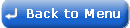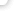4101. Why has the system has automatically calculated a student loan deduction even though the annual salary for this employee will not exceed the Student Loan threshold?. Student loan deductions are calculated at a specified % of any income earned in excess of the Student Loan annual threshold. This annual threshold is divided by 12 for a monthly pay period threshold and by 52 for a weekly pay period threshold. Deductions are non-cumulative, and are calculated and taken in each pay period in which the employee's income exceeds the threshold for the pay period. For example, assuming the monthly pay period threshold for student loan is of £1250 and the deduction rate for student loan is 9%. If the employee's salary this month is £1300, this is £50 above the threshold so the employee will incur a student loan deduction of 9% of £50.